# Cross Validation: The Right and Wrong Way¶

For a real-world example that showcases the pitfalls of improper cross validation, see this blog post.

## The scenario¶

You have 20 datapoints, each of which has 1,000,000 attributes. Each observation also has an associated $y$ value, and you are interested in whether a linear combination of a few attributes can be used to predict $y$. That is, you are looking for a model

$$y_i \sim \sum_j w_j x_{ij}$$

where most of the 1 million $w_j$ values are 0.

## The problem¶

Since there are so many more attributes than datapoints, the chance that a few attributes correlate with $y$ by pure coincidence is fairly high.

You kind of remember that cross-validation helps you detect over-fitting, but you're fuzzy on the details.

## The wrong way to cross-validate¶

• Determine a few attributes of X that correlate well with Y
• Use cross-validation to measure how well a linear fit to these attributes predicts y
In :
%matplotlib inline

import numpy as np
import matplotlib.pyplot as plt

from sklearn.feature_selection import SelectKBest, f_regression
from sklearn.cross_validation import cross_val_score, KFold
from sklearn.linear_model import LinearRegression


Let's make the dataset, and compute the y's with a "hidden" model that we are trying to recover

In :
def hidden_model(x):
#y is a linear combination of columns 5 and 10...
result = x[:, 5] + x[:, 10]
#... with a little noise
result += np.random.normal(0, .005, result.shape)
return result

def make_x(nobs):
return np.random.uniform(0, 3, (nobs, 10 ** 6))

x = make_x(20)
y = hidden_model(x)

print(x.shape)

(20, 1000000)


Find the 2 attributes in X that best correlate with y

In :
selector = SelectKBest(f_regression, k=2).fit(x, y)
best_features = np.where(selector.get_support())
print(best_features)

[341338 690135]


We know we are already in trouble -- we've selected 2 columns which correlate with Y by chance, but neither of which are columns 5 or 10 (the only 2 columns that actually have anything to do with y). We can look at the correlations between these columns and Y, and confirm they are pretty good (again, just a coincidence):

In :
for b in best_features:
plt.plot(x[:, b], y, 'o')
plt.title("Column %i" % b)
plt.xlabel("X")
plt.ylabel("Y")
plt.show()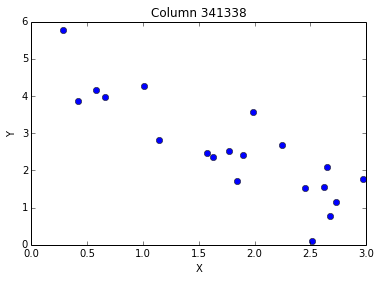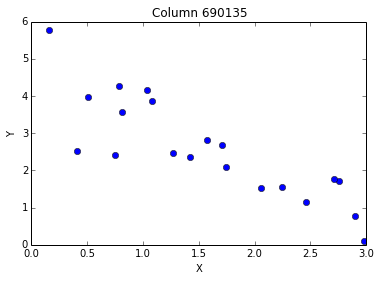A linear regression on the full data looks good. The "score" here is the $R^2$ score -- scores close to 1 imply a good fit.

In :
xt = x[:, best_features]
clf = LinearRegression().fit(xt, y)
print("Score is ", clf.score(xt, y))

Score is  0.839859389027

In :
yp = clf.predict(xt)
plt.plot(yp, y, 'o')
plt.plot(y, y, 'r-')
plt.xlabel("Predicted")
plt.ylabel("Observed")

Out:
<matplotlib.text.Text at 0x109e426d0>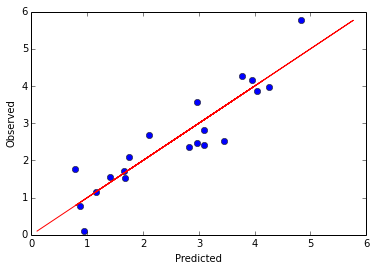We're worried about overfitting, and remember that cross-validation is supposed to detect this. Let's look at the average $R^2$ score, when performing 5-fold cross validation. It's not as good, but still not bad...

In :
cross_val_score(clf, xt, y, cv=5).mean()

Out:
0.61616795686754722

And even if we make some plots of the predicted and actual data at each cross-validation iteration, the model seems to predict the "independent" data pretty well...

In :
for train, test in KFold(len(y), 10):
xtrain, xtest, ytrain, ytest = xt[train], xt[test], y[train], y[test]

clf.fit(xtrain, ytrain)
yp = clf.predict(xtest)

plt.plot(yp, ytest, 'o')
plt.plot(ytest, ytest, 'r-')

plt.xlabel("Predicted")
plt.ylabel("Observed")

Out:
<matplotlib.text.Text at 0x109ec4810>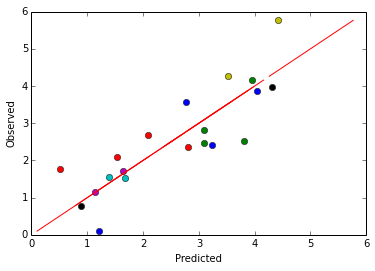But -- what if we generated some more data?

In :
x2 = make_x(100)
y2 = hidden_model(x2)
x2 = x2[:, best_features]

y2p = clf.predict(x2)

plt.plot(y2p, y2, 'o')
plt.plot(y2, y2, 'r-')
plt.xlabel("Predicted")
plt.ylabel("Observed")

Out:
[<matplotlib.lines.Line2D at 0x1136efa90>]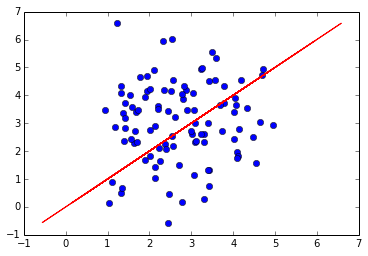Yikes -- there is no correlation at all! Cross-validation did not detect the overfitting, because we used the entire data to select "good" features.

## The right way to cross-validate¶

To prevent overfitting, we can't let any information about the full dataset leak into cross-validation. Thus, we must re-select good features in each cross-validation iteration

In :
scores = []

for train, test in KFold(len(y), n_folds=5):
xtrain, xtest, ytrain, ytest = x[train], x[test], y[train], y[test]

b = SelectKBest(f_regression, k=2)
b.fit(xtrain, ytrain)
xtrain = xtrain[:, b.get_support()]
xtest = xtest[:, b.get_support()]

clf.fit(xtrain, ytrain)
scores.append(clf.score(xtest, ytest))

yp = clf.predict(xtest)
plt.plot(yp, ytest, 'o')
plt.plot(ytest, ytest, 'r-')

plt.xlabel("Predicted")
plt.ylabel("Observed")

print("CV Score is ", np.mean(scores))

CV Score is  -1.64839183777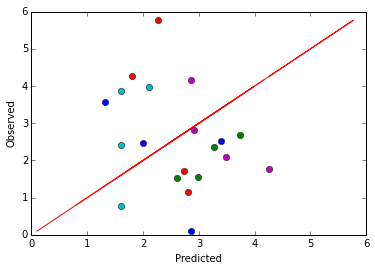Now cross-validation properly detects overfitting, by reporting a low average $R^2$ score and a plot that looks like noise. Of course, it doesn't help us actually discover the fact that columns 5 and 10 determine Y (this task is probably hopeless without more data) -- it just lets us know when our fitting approach isn't generalizing to new data.